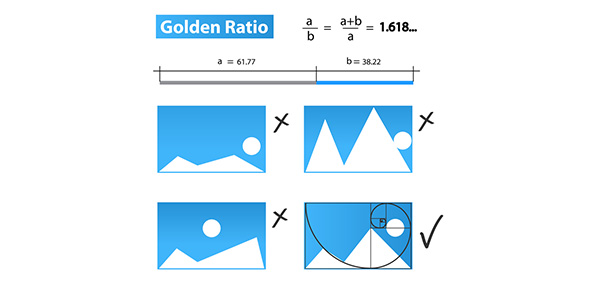# Ratios - Guided Practice Quiz

9 Questions | Attempts: 102
ShareSettingsRatios are forms of equations. They are formed by comparing two variables showing how many of each exists in the other. The variables are separated by use of colons. Test how good you are at forming ratios for different scenarios by taking the quiz below. All the best as you test yourself.

• 1.
Express each ratio as a fraction in simplest form.12 boys to 16 girls
• 2.
Express each ratio as a fraction in simplest form.24 out of 60 light bulbs
• 3.
Express each ratio as a fraction in simplest form.36 DVD's out of 84 DVD's
• 4.
Express each ratio as a fraction in simplest form.50 tiles to 25 tiles
• 5.
In Mr. Blackwell's class, 15 out of 24 students play sports. Express this ratio as a fraction in simplest form
• 6.
Express each ratio as a fraction in simplest form.3 pints to 4 quarts
• 7.
Express each ratio as a fraction in simplest form.2 pounds to 6 ounces
• 8.
Express each ratio as a fraction in simplest form.9 inches to 1 yard
• 9.
Express each ratio as a fraction in simplest form.6 gallons to 3 quarts

## Related TopicsBack to top
×

Wait!
Here's an interesting quiz for you.